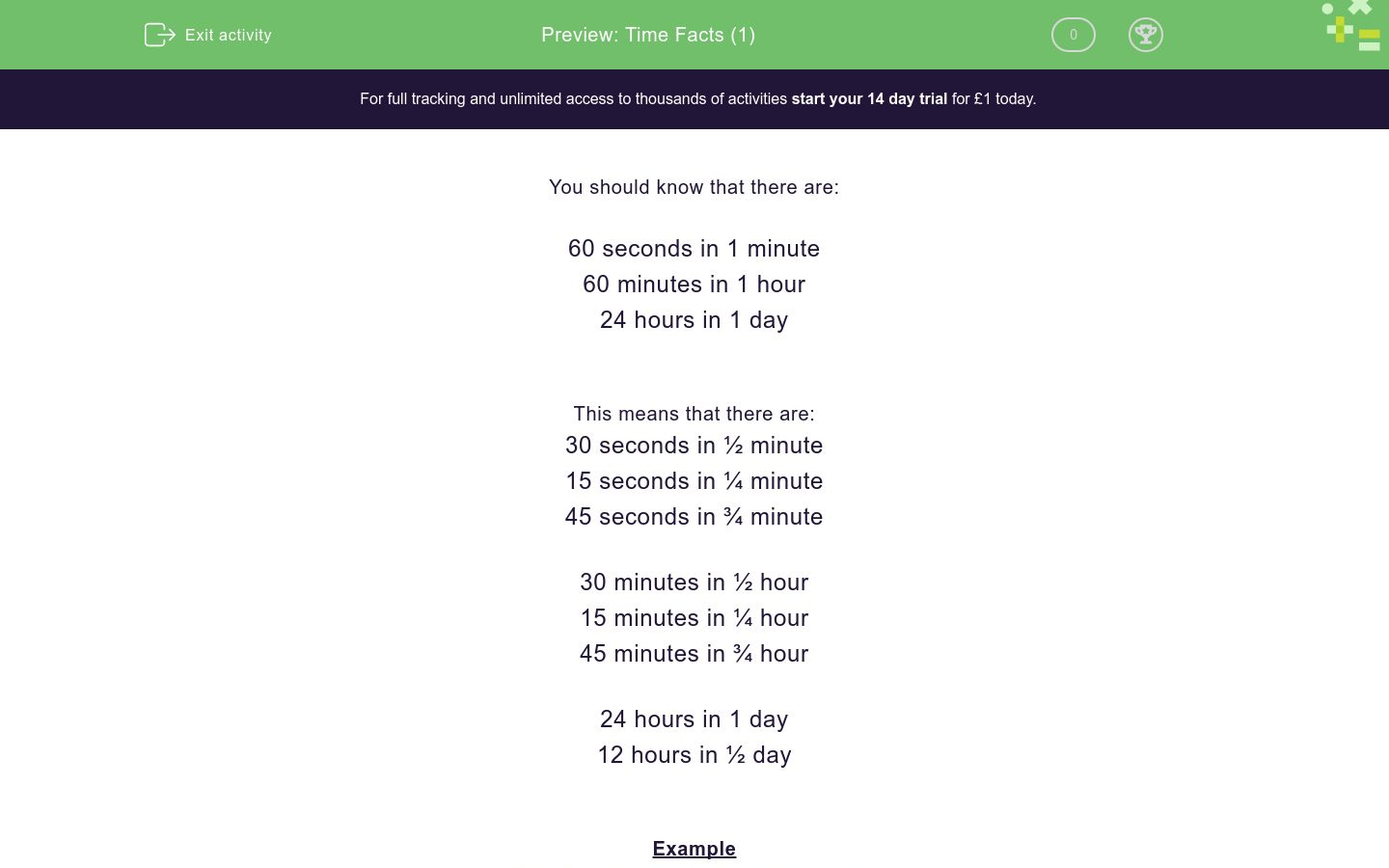# Time Facts (1)

In this worksheet, students must know how many seconds, minutes and hours there are in various time periods, including halves and quarters.Key stage:  KS 2

Curriculum topic:   Measurement

Curriculum subtopic:   Know Measurements of Time

Difficulty level:### QUESTION 1 of 10

You should know that there are:

60 seconds in 1 minute

60 minutes in 1 hour

24 hours in 1 day

This means that there are:

30 seconds in ½ minute

15 seconds in ¼ minute

45 seconds in ¾ minute

30 minutes in ½ hour

15 minutes in ¼ hour

45 minutes in ¾ hour

24 hours in 1 day

12 hours in ½ day

Example

Ahmed takes 3¼ minutes to eat his breakfast.

How many seconds is this?

1 minute = 60 seconds.

½ minute = 30 seconds.

¼ minute = 15 seconds.

3¼ minutes = 60 + 60 + 60 + 15 = 195 seconds.

Write the missing number below:

There areminutes in 3 hours.

Write the missing number below:

There areseconds in 5 minutes.

Write the missing number below:

There arehours in half a day.

Write the missing number below:

There areseconds in 1½ minutes.

Write the missing number below:

There areseconds in ¼ minute.

Choose the missing number below:

90 minutes is the same ashours.

2

Jack runs a marathon in 4 hours.

How many minutes is this?

(Just write the number.)

Ann starts painting a wall at 11:30 a.m. and finishes at 2:00 p.m.

How many minutes did she take?

150

90

930

Write the missing number below:

There areminutes in ¾ hour.

A tube train arrives at a station every 2¼ minutes.

How many seconds is this?

(Just write the number.)

• Question 1

Write the missing number below:

There areminutes in 3 hours.

180
EDDIE SAYS
60 minutes = 1 hour
3 × 60 = 180
• Question 2

Write the missing number below:

There areseconds in 5 minutes.

300
EDDIE SAYS
60 seconds = 1 minute
5 × 60 = 300
• Question 3

Write the missing number below:

There arehours in half a day.

12
EDDIE SAYS
24 hours = 1 day
24 ÷ 2 = 12
• Question 4

Write the missing number below:

There areseconds in 1½ minutes.

90
EDDIE SAYS
60 seconds = 1 minute
30 seconds = ½ minute
60 + 30 = 90
• Question 5

Write the missing number below:

There areseconds in ¼ minute.

15
EDDIE SAYS
60 seconds = 1 minute
30 seconds = ½ minute
15 seconds = ¼ minute
• Question 6

Choose the missing number below:

90 minutes is the same ashours.

EDDIE SAYS
60 minutes = 1 hour
30 minutes = ½ hour
• Question 7

Jack runs a marathon in 4 hours.

How many minutes is this?

(Just write the number.)

240
EDDIE SAYS
60 minutes = 1 hour
4 × 60 = 240 minutes
• Question 8

Ann starts painting a wall at 11:30 a.m. and finishes at 2:00 p.m.

How many minutes did she take?

150
EDDIE SAYS
She takes 2½ hours.
60 minutes = 1 hour
60 minutes = 1 hour
30 minutes = ½ hour
150 minutes = 2½ hours
• Question 9

Write the missing number below:

There areminutes in ¾ hour.

45
EDDIE SAYS
¼ hour = 15 mins so ¾ hour = 45 mins
• Question 10

A tube train arrives at a station every 2¼ minutes.

How many seconds is this?

(Just write the number.)

135
EDDIE SAYS
60 seconds = 1 minute
30 seconds = ½ minute
15 seconds = ¼ minute
60 + 60 + 15 = 135
---- OR ----

Sign up for a £1 trial so you can track and measure your child's progress on this activity.

### What is EdPlace?

We're your National Curriculum aligned online education content provider helping each child succeed in English, maths and science from year 1 to GCSE. With an EdPlace account you’ll be able to track and measure progress, helping each child achieve their best. We build confidence and attainment by personalising each child’s learning at a level that suits them.

Get started